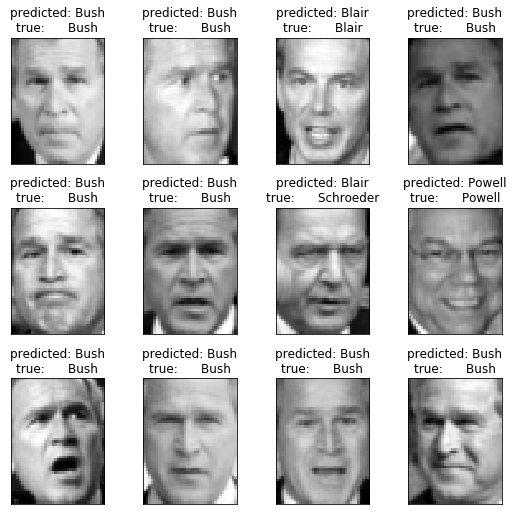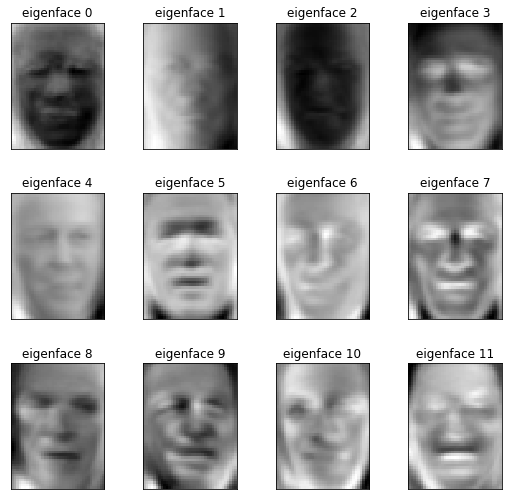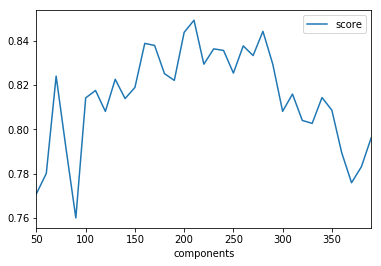# PCA Mini-Project¶

### Faces recognition example using eigenfaces and SVMs¶

This project is about reducing faces to a general form to be able to use this data to apply face recognition.

Note: The dataset used in this example is a preprocessed excerpt of the "Labeled Faces in the Wild", aka LFW_ Download (233MB). Original source.

In :
from time import time
import logging
import pylab as pl
import numpy as np

from sklearn.model_selection import train_test_split
from sklearn.datasets import fetch_lfw_people
from sklearn.model_selection import GridSearchCV
from sklearn.metrics import classification_report
from sklearn.metrics import confusion_matrix
from sklearn.decomposition import RandomizedPCA
from sklearn.decomposition import PCA
from sklearn.svm import SVC


In :
# Download the data, if not already on disk and load it as numpy arrays
lfw_people = fetch_lfw_people('data', min_faces_per_person=70, resize=0.4)

# introspect the images arrays to find the shapes (for plotting)
n_samples, h, w = lfw_people.images.shape
np.random.seed(42)

# for machine learning we use the data directly (as relative pixel
# position info is ignored by this model)
X = lfw_people.data
n_features = X.shape

# the label to predict is the id of the person
y = lfw_people.target
target_names = lfw_people.target_names
n_classes = target_names.shape

print("Total dataset size:")
print("n_samples: %d" % n_samples)
print("n_features: %d" % n_features)
print( "n_classes: %d" % n_classes)

Total dataset size:
n_samples: 1288
n_features: 1850
n_classes: 7


### Split into a training and testing set¶

In :
X_train, X_test, y_train, y_test = train_test_split(X, y, test_size=0.25, random_state=42)


## Compute PCA¶

We can now compute a PCA (eigenfaces) on the face dataset (treated as unlabeled dataset): unsupervised feature extraction / dimensionality reduction.

In :
n_components = 150

print( "Extracting the top %d eigenfaces from %d faces" % (n_components, X_train.shape) )
t0 = time()

# TODO: Create an instance of PCA, initializing with n_components=n_components and whiten=True
pca = PCA(n_components=n_components, whiten=True, svd_solver='randomized')

#TODO: pass the training dataset (X_train) to pca's 'fit()' method
pca = pca.fit(X_train)

print("done in %0.3fs" % (time() - t0))

Extracting the top 150 eigenfaces from 966 faces
done in 0.338s


Projecting the input data on the eigenfaces orthonormal basis

In :
eigenfaces = pca.components_.reshape((n_components, h, w))

t0 = time()
X_train_pca = pca.transform(X_train)
X_test_pca = pca.transform(X_test)
print("done in %0.3fs" % (time() - t0))

done in 0.032s


## Train a SVM classification model¶

Let's fit a SVM classifier to the training set. We'll use GridSearchCV to find a good set of parameters for the classifier.

In :
param_grid = {
'C': [1e3, 5e3, 1e4, 5e4, 1e5],
'gamma': [0.0001, 0.0005, 0.001, 0.005, 0.01, 0.1],
}

# for sklearn version 0.16 or prior, the class_weight parameter value is 'auto'
clf = GridSearchCV(SVC(kernel='rbf', class_weight='balanced'), param_grid)
clf = clf.fit(X_train_pca, y_train)

print("Best estimator found by grid search:")
print(clf.best_estimator_)

Best estimator found by grid search:
SVC(C=1000.0, cache_size=200, class_weight='balanced', coef0=0.0,
decision_function_shape='ovr', degree=3, gamma=0.001, kernel='rbf',
max_iter=-1, probability=False, random_state=None, shrinking=True,
tol=0.001, verbose=False)


## Evaluation of the model quality on the test set¶

#### 1. Classification Report¶

Now that we have the classifier trained, let's run it on the test dataset and qualitatively evaluate its results. Sklearn's classification_report shows some of the main classification metrics for each class.

In :
y_pred = clf.predict(X_test_pca)

print(classification_report(y_test, y_pred, target_names=target_names))

                   precision    recall  f1-score   support

Ariel Sharon       0.56      0.69      0.62        13
Colin Powell       0.74      0.87      0.80        60
Donald Rumsfeld       0.76      0.81      0.79        27
George W Bush       0.93      0.87      0.90       146
Gerhard Schroeder       0.76      0.76      0.76        25
Hugo Chavez       0.73      0.53      0.62        15
Tony Blair       0.88      0.83      0.86        36

avg / total       0.84      0.83      0.83       322



#### 2. Confusion Matrix¶

Another way to look at the performance of the classifier is by looking the confusion matrix. We can do that by simply invoking sklearn.metrics.confusion_matrix:

In :
print(confusion_matrix(y_test, y_pred, labels=range(n_classes)))

[[  9   0   3   1   0   0   0]
[  2  52   1   4   0   1   0]
[  4   0  22   1   0   0   0]
[  1  11   2 127   3   1   1]
[  0   2   0   1  19   1   2]
[  0   3   0   1   2   8   1]
[  0   2   1   2   1   0  30]]


#### 3. Plotting The Most Significant Eigenfaces¶

In :
def plot_gallery(images, titles, h, w, n_row=3, n_col=4):
"""Helper function to plot a gallery of portraits"""
pl.figure(figsize=(1.8 * n_col, 2.4 * n_row))
for i in range(n_row * n_col):
pl.subplot(n_row, n_col, i + 1)
pl.imshow(images[i].reshape((h, w)), cmap=pl.cm.gray)
pl.title(titles[i], size=12)
pl.xticks(())
pl.yticks(())

# plot the result of the prediction on a portion of the test set

def title(y_pred, y_test, target_names, i):
pred_name = target_names[y_pred[i]].rsplit(' ', 1)[-1]
true_name = target_names[y_test[i]].rsplit(' ', 1)[-1]
return ('predicted: %s\ntrue:      %s' % (pred_name, true_name))

prediction_titles = [title(y_pred, y_test, target_names, i)
for i in range(y_pred.shape)]

plot_gallery(X_test, prediction_titles, h, w)

pl.show()In :
eigenface_titles = ["eigenface %d" % i for i in range(eigenfaces.shape)]
plot_gallery(eigenfaces, eigenface_titles, h, w)

pl.show()## Explained Variance Of Each PC¶

We mentioned that PCA will order the principal components, with the first PC giving the direction of maximal variance, second PC has second-largest variance, and so on. How much of the variance is explained by the first principal component? The second?

In :
print(pca.components_)
print("This one explains {} variance.".format(pca.explained_variance_ratio_))

print(pca.components_)
print("This one explains {} variance.".format(pca.explained_variance_ratio_))

[-0.00685205 -0.00752694 -0.00918908 ..., -0.01433783 -0.01294188
-0.01188291]
This one explains 0.19346508383750916 variance.
[ 0.02304089  0.02155207  0.0227539  ..., -0.0440019  -0.04318583
-0.04226125]
This one explains 0.15116845071315765 variance.


## How Many PCs To Use?¶

In a multiclass classification problem like this one (more than 2 labels to apply), accuracy is a less-intuitive metric than in the 2-class case. Instead, a popular metric is the F1 score.

We’ll figure out for ourself whether a good classifier is characterized by a high or low F1 score. We’ll do this by varying the number of principal components and watching how the F1 score changes in response.

As you add more principal components as features for training your classifier, do you expect it to get better or worse performance?

I would say, that the more principal components you pick, the more the model overfits. If you pick just a few principal components the model would probably generalize too much. So we need to find the golden middle.

## F1 Score Vs. No. Of PCs Used¶

We're going to chnage n_components to the following values: [10, 15, 25, 50, 100, 250]. For each number of principal components, we note the F1 score for Ariel Sharon. (For 10 PCs, the plotting functions in the code will break, but we should be able to see the F1 scores.)

In :
clf = SVC(C=1000.0, cache_size=200, class_weight='balanced', coef0=0.0,
decision_function_shape='ovr', degree=3, gamma=0.001, kernel='rbf',
max_iter=-1, probability=False, random_state=None, shrinking=True,
tol=0.001, verbose=False)
for i in [10, 15, 25, 50, 100, 250,300,400]:
# TODO: Create an instance of PCA, initializing with n_components=n_components and whiten=True
pca = PCA(n_components=i, whiten=True, svd_solver='randomized')

#TODO: pass the training dataset (X_train) to pca's 'fit()' method
pca = pca.fit(X_train)

#eigenfaces = pca.components_.reshape((n_components, h, w))

X_train_pca = pca.transform(X_train)
X_test_pca = pca.transform(X_test)

clf.fit(X_train_pca, y_train)

y_pred = clf.predict(X_test_pca)

print("Taking {} components:".format(i))
print(classification_report(y_test, y_pred, target_names=target_names))

Taking 10 components:
precision    recall  f1-score   support

Ariel Sharon       0.13      0.38      0.20        13
Colin Powell       0.52      0.53      0.53        60
Donald Rumsfeld       0.29      0.41      0.34        27
George W Bush       0.75      0.38      0.50       146
Gerhard Schroeder       0.21      0.28      0.24        25
Hugo Chavez       0.15      0.47      0.23        15
Tony Blair       0.39      0.33      0.36        36

avg / total       0.54      0.40      0.43       322

Taking 15 components:
precision    recall  f1-score   support

Ariel Sharon       0.22      0.54      0.31        13
Colin Powell       0.61      0.65      0.63        60
Donald Rumsfeld       0.48      0.59      0.53        27
George W Bush       0.85      0.67      0.75       146
Gerhard Schroeder       0.40      0.56      0.47        25
Hugo Chavez       0.38      0.40      0.39        15
Tony Blair       0.56      0.42      0.48        36

avg / total       0.66      0.61      0.62       322

Taking 25 components:
precision    recall  f1-score   support

Ariel Sharon       0.40      0.62      0.48        13
Colin Powell       0.74      0.85      0.79        60
Donald Rumsfeld       0.48      0.48      0.48        27
George W Bush       0.93      0.79      0.86       146
Gerhard Schroeder       0.59      0.80      0.68        25
Hugo Chavez       0.62      0.53      0.57        15
Tony Blair       0.64      0.64      0.64        36

avg / total       0.76      0.74      0.75       322

Taking 50 components:
precision    recall  f1-score   support

Ariel Sharon       0.50      0.69      0.58        13
Colin Powell       0.78      0.83      0.81        60
Donald Rumsfeld       0.54      0.56      0.55        27
George W Bush       0.88      0.84      0.86       146
Gerhard Schroeder       0.67      0.72      0.69        25
Hugo Chavez       0.70      0.47      0.56        15
Tony Blair       0.69      0.69      0.69        36

avg / total       0.77      0.77      0.77       322

Taking 100 components:
precision    recall  f1-score   support

Ariel Sharon       0.53      0.62      0.57        13
Colin Powell       0.75      0.85      0.80        60
Donald Rumsfeld       0.56      0.67      0.61        27
George W Bush       0.90      0.87      0.89       146
Gerhard Schroeder       0.83      0.80      0.82        25
Hugo Chavez       0.90      0.60      0.72        15
Tony Blair       0.78      0.69      0.74        36

avg / total       0.81      0.80      0.80       322

Taking 250 components:
precision    recall  f1-score   support

Ariel Sharon       0.56      0.69      0.62        13
Colin Powell       0.73      0.92      0.81        60
Donald Rumsfeld       0.82      0.67      0.73        27
George W Bush       0.92      0.91      0.92       146
Gerhard Schroeder       0.76      0.64      0.70        25
Hugo Chavez       0.80      0.53      0.64        15
Tony Blair       0.82      0.78      0.80        36

avg / total       0.84      0.83      0.83       322

Taking 300 components:
precision    recall  f1-score   support

Ariel Sharon       0.62      0.77      0.69        13
Colin Powell       0.75      0.93      0.83        60
Donald Rumsfeld       0.95      0.67      0.78        27
George W Bush       0.89      0.91      0.90       146
Gerhard Schroeder       0.85      0.68      0.76        25
Hugo Chavez       0.80      0.53      0.64        15
Tony Blair       0.84      0.75      0.79        36

avg / total       0.84      0.84      0.83       322

Taking 400 components:
precision    recall  f1-score   support

Ariel Sharon       0.58      0.85      0.69        13
Colin Powell       0.67      0.92      0.77        60
Donald Rumsfeld       1.00      0.59      0.74        27
George W Bush       0.86      0.88      0.87       146
Gerhard Schroeder       0.87      0.52      0.65        25
Hugo Chavez       0.80      0.53      0.64        15
Tony Blair       0.81      0.72      0.76        36

avg / total       0.82      0.80      0.80       322



Let's plot how the f1-score develops for each number of components in the range 50 to 400:

In :
from sklearn.metrics import f1_score
import pandas as pd

df_f1 = []

for i in range(50,400,10):
# TODO: Create an instance of PCA, initializing with n_components=n_components and whiten=True
pca = PCA(n_components=i, whiten=True, svd_solver='randomized')

#TODO: pass the training dataset (X_train) to pca's 'fit()' method
pca = pca.fit(X_train)

#eigenfaces = pca.components_.reshape((n_components, h, w))

X_train_pca = pca.transform(X_train)
X_test_pca = pca.transform(X_test)

clf.fit(X_train_pca, y_train)

y_pred = clf.predict(X_test_pca)

print("Taking {} components".format(i))
df_f1.append({"components":i,"score":f1_score(y_test, y_pred,average='weighted')})
df_f1 = pd.DataFrame(df_f1)

Taking 50 components
Taking 60 components
Taking 70 components
Taking 80 components
Taking 90 components
Taking 100 components
Taking 110 components
Taking 120 components
Taking 130 components
Taking 140 components
Taking 150 components
Taking 160 components
Taking 170 components
Taking 180 components
Taking 190 components
Taking 200 components
Taking 210 components
Taking 220 components
Taking 230 components
Taking 240 components
Taking 250 components
Taking 260 components
Taking 270 components
Taking 280 components
Taking 290 components
Taking 300 components
Taking 310 components
Taking 320 components
Taking 330 components
Taking 340 components
Taking 350 components
Taking 360 components
Taking 370 components
Taking 380 components
Taking 390 components

In :
df_f1.plot(x=["components"], y=["score"])

Out:
<matplotlib.axes._subplots.AxesSubplot at 0x7f30ba811d30>